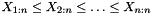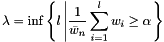#Boost C++ Libraries

...one of the most highly regarded and expertly designed C++ library projects in the world.

This is the documentation for an old version of boost. Click here for the latest Boost documentation.

## Struct template weighted_tail_quantile_impl

boost::accumulators::impl::weighted_tail_quantile_impl — Tail quantile estimation based on order statistics of weighted samples (for both left and right tails)

## Synopsis

```// In header: <boost/accumulators/statistics/weighted_tail_quantile.hpp>

template<typename Sample, typename Weight, typename LeftRight>
struct weighted_tail_quantile_impl {
// types
typedef numeric::functional::fdiv< Weight, std::size_t >::result_type float_type;
typedef Sample                                                        result_type;

// construct/copy/destruct
weighted_tail_quantile_impl(dont_care);

// public member functions
template<typename Args> result_type result(Args const &) const;
};```

## Description

An estimatorof tail quantiles with levelbased on order statisticsof weighted samples are given by(left tail) and(right tail), where

Equation 1.42.and

Equation 1.43.being the number of samples andthe sum of all weights.

### `weighted_tail_quantile_impl` public construct/copy/destruct

1. `weighted_tail_quantile_impl(dont_care);`

### `weighted_tail_quantile_impl` public member functions

1. `template<typename Args> result_type result(Args const & args) const;`